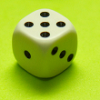Search by Topic

Resources tagged with Dice similar to Tug Harder!:

Filter by: Content type:
Age range:
Challenge level:

There are 31 results

Broad Topics > Mathematics Tools > Dice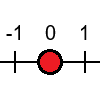Tug Harder!

Age 7 to 11 Challenge Level:

In this game, you can add, subtract, multiply or divide the numbers on the dice. Which will you do so that you get to the end of the number line first?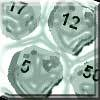Asteroid Blast

Age 7 to 11 Challenge Level:

A game for 2 people. Use your skills of addition, subtraction, multiplication and division to blast the asteroids.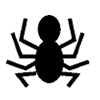KS2 Teacher Playing with Dice Collection

Age 7 to 11 Challenge Level:First Connect Three

Age 7 to 14 Challenge Level:

Add or subtract the two numbers on the spinners and try to complete a row of three. Are there some numbers that are good to aim for?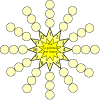The Twelve Pointed Star Game

Age 7 to 11 Challenge Level:

Have a go at this game which involves throwing two dice and adding their totals. Where should you place your counters to be more likely to win?Dice and Spinner Numbers

Age 7 to 11 Challenge Level:

If you had any number of ordinary dice, what are the possible ways of making their totals 6? What would the product of the dice be each time?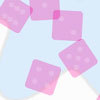Odds or Sixes?

Age 7 to 11 Challenge Level:

Use the interactivity or play this dice game yourself. How could you make it fair?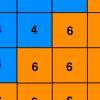Six Ten Total

Age 7 to 11 Challenge Level:

This challenge combines addition, multiplication, perseverance and even proof.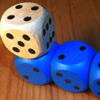The Dice Train

Age 7 to 11 Challenge Level:

This dice train has been made using specific rules. How many different trains can you make?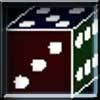The Path of the Dice

Age 7 to 11 Challenge Level:

A game for 1 person. Can you work out how the dice must be rolled from the start position to the finish? Play on line.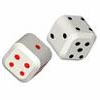Tricky Track

Age 7 to 11 Challenge Level:

In this game you throw two dice and find their total, then move the appropriate counter to the right. Which counter reaches the purple box first?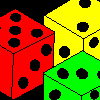Age 7 to 11 Challenge Level:

Three dice are placed in a row. Find a way to turn each one so that the three numbers on top of the dice total the same as the three numbers on the front of the dice. Can you find all the ways to do. . . .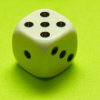A Bit of a Dicey Problem

Age 7 to 11 Challenge Level:

When you throw two regular, six-faced dice you have more chance of getting one particular result than any other. What result would that be? Why is this?Scoring with Dice

Age 7 to 11 Challenge Level:

I throw three dice and get 5, 3 and 2. Add the scores on the three dice. What do you get? Now multiply the scores. What do you notice?Three Dice

Age 7 to 11 Challenge Level:

Investigate the sum of the numbers on the top and bottom faces of a line of three dice. What do you notice?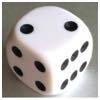Roll over the Dice

Age 7 to 11 Challenge Level:

Watch this video to see how to roll the dice. Now it's your turn! What do you notice about the dice numbers you have recorded?Dice Stairs

Age 7 to 11 Challenge Level:

Can you make dice stairs using the rules stated? How do you know you have all the possible stairs?How Many Dice?

Age 11 to 14 Challenge Level:

A standard die has the numbers 1, 2 and 3 are opposite 6, 5 and 4 respectively so that opposite faces add to 7? If you make standard dice by writing 1, 2, 3, 4, 5, 6 on blank cubes you will find. . . .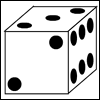Right or Left?

Age 7 to 11 Challenge Level:

Which of these dice are right-handed and which are left-handed?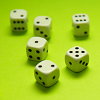Roll These Dice

Age 7 to 11 Challenge Level:

Roll two red dice and a green dice. Add the two numbers on the red dice and take away the number on the green. What are all the different possible answers?Dice, Routes and Pathways

Age 5 to 14

This article for teachers discusses examples of problems in which there is no obvious method but in which children can be encouraged to think deeply about the context and extend their ability to. . . .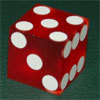Taking a Die for a Walk

Age 5 to 11 Challenge Level:

Investigate the numbers that come up on a die as you roll it in the direction of north, south, east and west, without going over the path it's already made.Round the Four Dice

Age 7 to 11 Challenge Level:

This activity involves rounding four-digit numbers to the nearest thousand.Round the Dice Decimals 2

Age 7 to 11 Challenge Level:

What happens when you round these numbers to the nearest whole number?Dicing with Numbers

Age 11 to 14 Challenge Level:

In how many ways can you arrange three dice side by side on a surface so that the sum of the numbers on each of the four faces (top, bottom, front and back) is equal?Spinners Environment

Age 5 to 18 Challenge Level:

A tool for generating random integers.Troublesome Dice

Age 11 to 14 Challenge Level:

When dice land edge-up, we usually roll again. But what if we didn't...?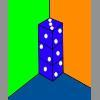Dice in a Corner

Age 7 to 11 Challenge Level:

How could you arrange at least two dice in a stack so that the total of the visible spots is 18?Round the Dice Decimals 1

Age 7 to 11 Challenge Level:

Use two dice to generate two numbers with one decimal place. What happens when you round these numbers to the nearest whole number?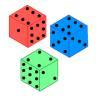Non-transitive Dice

Age 11 to 14 Challenge Level:

Alison and Charlie are playing a game. Charlie wants to go first so Alison lets him. Was that such a good idea?